# A Face Recognition Algorithm Based on Optimal Feature Selection

A Face Recognition Algorithm Based on Optimal Feature Selection
Image and Network Investigation Department, Railway Police College, Zhengzhou 450000, China

School of Intelligent Engineering, Zhengzhou University of Aeronautics, Zhengzhou 450000, China

Corresponding Author Email:
wangdan612@163.com
Page:
105-109
|
DOI:
https://doi.org/10.18280/ria.330204
10 January 2019
|
Accepted:
6 March 2019
|
Published:
25 August 2019
| Citation

OPEN ACCESS

Abstract:

To achieve accurate and robust face recognition, this paper designs a face recognition algorithm based on optimal feature selection. The algorithm is denoted as GRA-LSSVM, because it integrates grey relational analysis (GRA) with least squares support vector machine (LSSVM). Firstly, the target face image was segmented into several subblocks. Next, the global features of the face were extracted from each subblock by kernel principal component analysis (PCA). After that, the GRA algorithm was introduced to determine the features that contribute greatly to face recognition. These features were integrated into an eigenvector. Finally, a face classifier by the LSSVM based on the “one-to-many” principle, and simulated with multiple face databases. The simulation shows the GRA-LSSVM derived the optimal feature subset for face recognition, and thus outperformed other face recognition algorithms in accuracy and speed. The research provides an effective and advanced method for face recognition.

Keywords:

face recognition, feature selection, grey relational analysis (GRA), face classifier, recognition speed

1. Introduction

Among many biology identification technologies, the human face features contactless, uniqueness and low responses to environmental. As the most commonly used pattern recognition method, face recognition has quite broad practical application value in the fields of video monitoring and identity authentication. In practices, the features mainly describe the classes of faces, and such description constrains how well the face recognition effects are. For this purpose, it is the focus of current study on how to select the optimal face features to establish the face recognition algorithm [1-3].

There are local and global features on the face. Among them, the local features also include texture, LBP, color, etc. [4-6], which are used to depict the facial details; while the global features are used to portray overall information of face, for instance, the principal component analysis (PCA), the kernel PCA, etc., can extract facial features. Both global or local features can only describe partial face and capture fragments of face information, so that it is impossible for them to fully characterize the facial information [7-9]. To eliminate the restriction of single local or global features, some scholars have proposed a face recognition algorithm based on combined features, which extracts and combines both local features and global features from the face, enables a higher face recognition rate than that based on single local features or global features . However, these algorithms merely combine local or global features, resulting in the surge of the face features, increased computational complexity of face recognition, and poor timeliness of face recognition; while the combination of multiple features interfere with each other to a certain extent, increases the feature redundancy, and sometimes the face recognition precision gets worse. For this purpose, some scholars have proposed that the robust PCA merges local and global features to reduce the number of face recognition features. However, this method is easy to undermine the relationship between features, and the extracted features may be poorly interpretable [11-13]. Grey relational analysis (GRA) is an algorithm used for analyzing the relationship between variables. It enables to describe the relationship between variables, fuse and extract facial features, and pick out features important for face recognition, while filtering out those features useless or unimportant for face recognition, so that it can improve the face recognition rate . In the face recognition modeling process, the face classifier is also very important to play a key effect on the face recognition precision. Support Vector Machine (SVM), as the most commonly used face recognition classification algorithm, has quite redundant training time that affects face recognition efficiency. The least square support vector machine (LSSVM) improves the standard SVM to streamline and expedite the training process, and provides a new classifier for face recognition .

To fill the gaps of the current face recognition algorithms to improve the face recognition effect, a face recognition algorithm based on optimal feature selection (GRA-LSSVM) is designed and tested with the standard face databases for its availability and superiority.

2. Framework of Fave Recognition Alhorithm Based on Optimal Feature Selection

The face recognition algorithm based on optimal feature selection works in the following principle: first, denoise the face image, that is, obviate the interference of noise in the extraction of subsequent features. Then separately extract the local and global features from the face, and select those features that contribute the most to the face recognition results with the GRA algorithm. These features make up appropriate eigenvectors. Lastly, the least squares SVM is used to establish the classifier for face recognition based on the “one-to-many” principle, as shown in Figure 1.

## 1.jpgFigure 1. The work framework of face recognition algorithm

3. Design of Face Recognition Alorithm

3.1 Denoise face image

When collecting the face images, subject to illumination, operator technology, and acquisition equipment, the original faces available are inevitably interfered in the form of noise. For this, it is better to eliminate these interferences among extracted facial features, namely, by the face denoising process. A Gabor filter is sued to denoise the face. The Gabor filter with vector μ and scale v can be described as:

$\Psi_{u, v}(z)=\frac{\left\|k_{u, v}\right\|^{2}}{\delta^{2}} e^{\left(-\left\|k_{u,v}\right\|^{2} \|z||^{2} / 2 \delta^{2}\right)}\left[e^{i k_{u, v} z}-e^{-\delta^{2} / 2}\right]$      (1)

where, z=(x,y) represents the pixel position of the face; ku,v represents the working frequency of the Gabor filter on the vector (μ,v); δ represents the bandwidth of the Gabor filter.

A number of Gabor filters are combined in series for face denoising operations. Then these filter coefficients can be expressed as:

$\chi=\left(\boldsymbol{a}_{0,0}^{(\rho)^{T}} \boldsymbol{a}_{0,1}^{(\rho)^{T}}, \cdots \boldsymbol{a}_{0,7}^{(\rho)^{T}} ; \boldsymbol{a}_{1,0}^{(\rho)^{T}} \cdots \boldsymbol{a}_{4,7}^{(\rho)^{T}}\right)$      (2)

3.2 Extract facial features

(1) Local features. In the face recognition process, local features are rather important, and mainly used to describe the nose, the eyes and other organs of the face.

## 2.jpg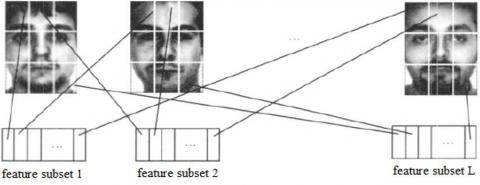Figure 2. Principle of face local feature extraction

There is a total of M classes of faces, a total of N face images, each face image has W1×W2 pixels. They make up a set of

faces: Train=(x1,x2,…,xN)W1W2×N, then the local features of the face may be extracted by the following steps:

Step1: divide each face image evenly to get L sub-images.

Step 2: Extract the features of each subblock, and the subblock’ features in the same position constitute a feature subset, then it is possible to create L feature subsets: train1, train2, …, trainl. The specific principle is shown in Figure 2.

(2) Global features. The KPCA is used to extract the global features of the face, assume the set of the face samples is X={x1, x2, …, xm}, then the following covariance matrix can be established:

$C=\frac{1}{M} \sum_{j-1}^{M} \phi\left(x_{j}\right) \phi\left(x_{j}\right)^{T}$    (3)

where, ϕ represents the mapping, and $\sum_{k-1}^{M} \phi\left(x_{k}\right)=0$.

Decompose C, then λv=Cv, and λ≥0 is true, where v represents the eigenvector used to describe the space composed of ϕ(x1), ϕ(x2), , ϕ(xm), then it follows that

$\lambda\left(\phi\left(x_{k}\right), v^{r}\right)=\left\langle\phi\left(x_{k}\right), C v^{r}\right\rangle, k=1,2, \cdots, M$        (4)

as vr is a linear combination of ϕ(x), it can be expressed as

$v^{r}=\sum_{i=1}^{M} c_{i}^{r} \phi\left(x_{i}\right)$    (5)

assume kij=⟨ϕ(xi),ϕ(xj)⟩, combine the above Formulas, it follows that

$M \lambda^{r} c^{r}=K c^{r}$        (6)

A eigenvector greater than zero is represented as cp, cp+1, ⋯, cM, normalize cr to produce: cr,cr=1, the projection of ϕ(x) on cr can be described as

$\phi\left(x_{i}\right)=\phi\left(x_{i}\right)-\frac{1}{M} \sum_{i=1}^{M} \phi\left(x_{i}\right)$    (7)

The principal component of ϕ(x) is projected to generate a new eigenvector: g(x)=[g1(x),g2(x),⋯,gl(x)]T, and the dot product operation can be implemented with K1(xi,x)=⟨ϕ(xi),ϕ(x)⟩, then:

$g(x)=\left\langle v^{r}, \phi(x)\right\rangle=\sum_{i=1}^{M} c^{r} K_{1}\left(x_{i}, x\right)$      (8)

The global features of face are extracted by the following way:

Step1: extract the features of the set of face samples by the KPCA to generate the projection matrix Wpca.

Step2: For all faces, the global eigenvectors of face recognition will be available based on Wpca projection: trainx=(x1,x2,…,xN)k2×N.

3.3 Select the optimal features by the GRA algorithm

(1) Assume the sequence composed of the sets of face features is Xi=(xi(1), xi(2), ⋯, xi(n)), i=1, 2, ⋯, m.

(2) Dimensionless processing is performed on Xi=(xi(1), xi(2), ⋯, xi(n)), i=1, 2, ⋯, m, after the initial value is available, select a set of reference objects X0'=(x0'(1), x0'(2),⋯, x0'(n)), i=1, 2, ⋯, m, and make up a sequence in relation to other sequences.

(3) Calculate the difference sequence based on Δi(k)=|x0'(k)-xi'(k)|, the maximum and minimum differences are then obtained: $M=\max _{i} \max _{k} \Delta_{i}(k)$ and $m=\min _{i} \min _{k} \Delta_{i}(k)$.

(4) Calculate the grey relational coefficient ri(k).

$r_{i}(k)=\frac{m+\xi M}{\Delta_{i}(k)+\xi M}$    (9)

where, ξ is resolution coefficient, k=1, 2, ⋯, n; i=1, 2, ⋯, m.

(5) Based on relational coefficient and weight, the gray comprehensive relationship degree is available, as shown below:

$r_{i}=\sum_{k=1}^{n} w_{i} r_{i}(k)$       (10)

where, wi represents the weight.

The GRA algorithm is used to estimate the relationship degree between the two features, eliminate redundant features to obtain the subset of optimal features.

3.4 Create the face classifier

Assume the set of samples for face recognition is (xi, yi), i=1, 2, …, n, where xi represents the face features; yi represents the types of faces, then such a functional expression can be available:

$f(x)=w^{T} \varphi(x)+b$    (11)

Based on the principle of structural risk minimization, we can obtain

$\min _{w, b, e} J(w, e)=\frac{1}{2} W^{T} W+\frac{\gamma}{2} \sum_{i=1}^{n} e_{i}^{2}$

s.t.

$y_{i}=w^{T} \varphi(x)+b+e_{i}, i=1,2, \cdots, l$       (12)

where, γ represents the regularization parameter of LSSVM.

After introducing the Lagrangian multiplier αi, it follows that

$L(w, b, e, \alpha)=J(w, e)-\sum_{i=1}^{l} \alpha_{i}\left(w^{T} \varphi\left(x_{i}\right)+b+e_{i}-y_{i}\right)$     (13)

Based on the KKT, then

$\left[\begin{array}{cc}{0} & {e 1^{T}} \\ {e 1} & {Q+\gamma^{-1} I}\end{array}\right]\left[\begin{array}{l}{b} \\ {a}\end{array}\right]=\left[\begin{array}{l}{0} \\ {y}\end{array}\right]$     (14)

and

$\left\{\begin{array}{l}{y=\left(y_{1}, y_{2}, \cdots, y_{l}\right)^{T}} \\ {e 1=(1,1, \cdots, 1)^{T}} \\ {a=\left(a_{1}, a_{2}, \cdots, a_{l}\right)^{T}}\end{array}\right.$     (15)

The classification function of the LSSVM is established based on the radial basis function, as follows:

$K\left(x_{i}, x_{j}\right)=\exp \left(-\frac{\left\|x_{i}-x_{j}\right\|^{2}}{2 \sigma^{2}}\right)$      (16)

where, σ is the width of radial basis function.

Since the face recognition is a multi-classification problem, and the LSSVM aims at two classification problems, the face classifier is established in the "one-to-many" form, as shown in Figure 3.

## 3.jpg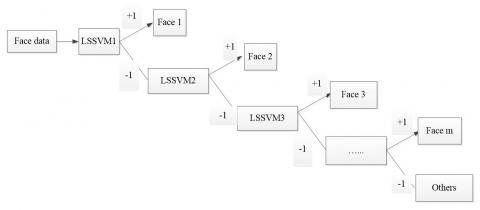Figure 3. Structure of face classifier

4. Simulation Test

4.1 Test object

To analyze the face recognition effect based on the GRA-LSSVM, the MATLAB 2014A is used as the test environment and the current 4 classic face databases as test objects.

## 4a.jpg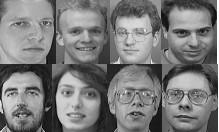(a) ORL faces

## 4b.jpg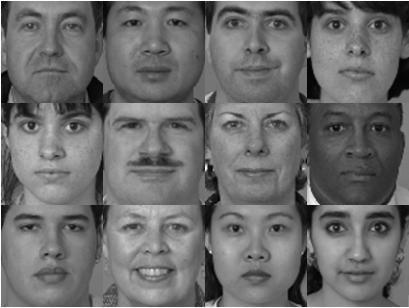(b) FERET faces

## 4c.jpg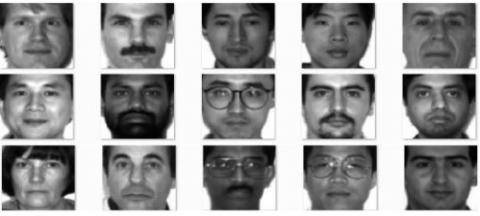(c) Yale B faces

## 4d.jpg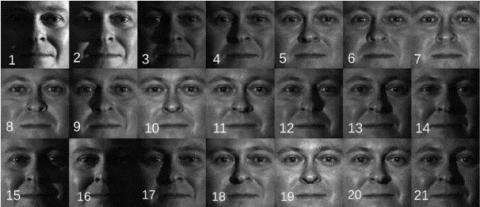(d) PIE faces

Figure 4. Face database

(1) the ORL database contains the faces of 40 people, each has 10 faces. Face acquisition environment is more ideal, and some faces are shown in Figure 4(a); (2) the FERET database contains the faces from 200 people, each has 7 faces collected subject to poses, expressions, illumination and other factors. Randomly selected faces are shown in Figure 4(b); (3) Yale B database contains the faces from 38 people, each has 64 faces. Under different illumination conditions, some faces are shown in Figure 4(c); (4) the PIE face database contains the faces of 65 people, each has 21 faces subject to illumination and expression changes. Some faces are shown in Figure 4(d). The normalization operation is performed on all faces to turn their size into 192×l68. The training and test samples are about 3:1, and the face recognition rate is:

$R(\%)=\frac{N_{r i g h t}}{N_{t e s t}} \times 100 \%$     (17)

where, Nright is the number of faces correctly recognized; Ntest is the total number of faces.

4.2 Test results and analysis

Selecting local features and LSSVM face recognition algorithm (LSSVM1), global features and LSSVM face recognition algorithm (LSSVM2), simple features and LSSVM face recognition algorithm (LSSVM3), a test is conducted for compare them, see Table 1 for average face recognition rates and the recognition time durations. Comparing the test results of Table 2, it is concluded that:

Table 1. Comparison of face recognition rate with different algorithms

 Arithmetic Face recognition rate rate/% ORL FERET Yale B PIE LSSVM1 91.23 86.35 94.17 79.30 LSSVM2 92.47 89.16 95.69 85.59 LSSVM3 93.67 91.11 97.31 85.57 GRA-LSSVM 94.12 90.93 98.09 89.98

Table 2. Comparison of face recognition time with different algorithms

 Arithmetic Average face recognition time /ms ORL FERET Yale B PIE LSSVM1 2.75 3.58 4.17 3.46 LSSVM2 3.42 4.65 4.02 4.13 LSSVM3 11.47 11.91 12.35 15.47 GRA-LSSVM 3.96 5.74 6.43 5.21

(1) With single local features or global features, the face recognition algorithm has the minimum recognition rate. It means that its false recognition rate is very high, so that it is impossible to correctly identify all faces since single features can only be partial features difficult to portray face class. However, face recognition with single features can consume short time on average, and the calculation complexity is higher. Due to low recognition rate, it cannot be applied to the actual environment.

(2) Compared with single local feature or global feature face recognition algorithm, the face recognition effect of LSSVM3 gets improved in that it reduces the false recognition rate. This is because the simple combination features can describe face class from multiple angles, and a better face recognition model is created to improve the face recognition precision. However, it greatly extends the face recognition time at a low face recognition efficiency, failing to reach the face recognition real-time performance highly required. In view of these defects, it has little application value in practices.

(3) Unlike the above face recognition algorithms, the GRA-LSSVM greatly improves the face recognition rate; reduces the average face recognition time to be significantly less than the simple combination recognition algorithm, optimizes the face recognition efficiency, because the GRA algorithm enables active merge on the facial features to extract better ones, filters out useless and redundant features. A better face classifier is established to realize the higher application value.

5. Conclusion

In order to improve face recognition rate, a GRA-LSSVM-based face recognition algorithm is proposed for feature selection in the face recognition process. First, the face is denoised and the local and global features are extracted from face. Next, the GRA algorithm can be used to select the optimal features and reduce the face calculation complexity, then the classifier is created for face recognition using the LSSVM, which conduct the simulation test with multiple face data. The results show that GRA-LSSVM-based face recognition rate has been greatly improved, and the face recognition time is less than that consumed by some combination feature algorithms. Face recognition has strong timeliness and will have a broad application prospect.

Acknowledgment

This work was supported in part by the National Natural Science Foundation of China under Grant 61801435, in part by the Project funded by China Postdoctoral Science Foundation under Grant 2018M633733, in part by the Scientific and Technological Key Project of Henan Province under Grant 192102210246, in part by the Scientific Key Research Project of Henan Province for Colleges and Universities under Grand 19A510024.

References

 Zhao, W., Chellappa, R., Phillips, P.J., Rosenfeld, A. (2009). Face recognition a literature survey. ACM Computing Surveys, 35(4): 399-458.

 Adana, Y., Moses, Y., Ullman, S. (2011). Face recognition: The problem of compensating for changes in illumination direction. IEEE Transactions on Pattern Analysis and Machine Intelligence, 19(7): 721-732. https://doi.org/10.1007/3-540-57956-7_33

 Benkaddour, M.K., Bounoua, A. (2017). Feature extraction and classification using deep convolutional neural networks, PCA and SVC for face recognition. Traitement du Signal, 34(1-2): 77-91. https://doi.org/10.3166/TS.34.77-91

 Oh, S.H., Kim, G.W., Lim, K.S. (2018). Compact deep learned feature-based face recognition for visual Internet of things. Journal of Supercomputing, 74(12): 6729-6741. https://doi.org/10.1007/s11227-017-2198-0

 Kumar, S., Singh, S., Kumar, J. (2018). Live detection of face using machine learning with multi-feature method. Wireless Personal Communications, 103(3): 2353-2375. https://doi.org/10.1007/s11277-018-5913-0

 Ding, Z.C., Mao, Q.R., Zhang, Y.Z., Wang, M.C. (2016). Face expression recognition method based on discriminative multi-features joint sparse representation. Journal of Chinese Computer Systems, 36(12): 2775-2779. http://xwxt.sict.ac.cn/EN/Y2016/V37/I12/2775

 Ghorai, M., Samanta, S., Mandal, S., Chanda, B. (2019). Multiple pyramids based image inpainting using local patch statistics and steering kernel feature. IEEE Transactions on Image Processing, 28(11): 5495-5509. https://doi.org/10.1109/TIP.2019.2920528

 Hu, Z.P., Peng, Y., Zhao, S.H. (2015). Sparse representation with weighted fusion of local based non-minimum square error and global for face recognition under occlusion condition. Pattern Recognition and Artificial Intelligence, 28(7): 633-640.

 Xu, Y., Zhong, A.N., Yang. J., Zhang, D. (2011). LPP solution schemes for use with face recognition. Pattern Recognition, 43(12): 4165-4176. https://doi.org/10.1016/j.patcog.2010.06.016

 Yang, G.L., Feng, Y.Q., Lu, H.R. (2015). Face recognition with varying illumination and occlusion based on sparse error of low rank representation. Computer Engineering & Science, 37(9): 1742-1749.

 Hsieh, P.C., Tung, P.C. (2009). A novel hybrid approach based on sub-pattern technique and whitened PCA for face recognition. Pattern Recognition, 42(5): 978-984. https://doi.org/10.1016/j.patcog.2008.09.024

 Yang, Z.J., Liu, C.C., Gu, X.J., Zhu, J. (2013). Probabilistic classification preserving projections and its application to face recognition. Journal of Nanjing University of Science and Technology, 37(1): 7-11.

 Li, G., Li, W.H. (2013). Face occlusion recognition based on MEBML. Journal of Jilin University Engineering and Technology Edition, 35(1): 171-184.

 Yang, W., Sun, H., Zheng, L.H., Zhang, Y., Li, M.Z. (2013). Grey analysis of NIR spectra and prediction of nitrogen content in jujube leaves. Spectroscopy and Spectral Analysis, 33(11): 3083-3087.

 Liu, Q.C., Shi, R.H., Wang, G.C., Mu, W.W. (2014). Study on intrusion detection algorithm based on rough set theory and improved LSSVM. Computer Engineering and Applications, 50(2): 99-102.